# Maths - Fibre Bundle

Fibres and fibre bundles are an important mathematical structure that occurs across many branches of maths. Therefore there are many ways to approach this subject:

## Fibre Bundle as Dependent Type

In type theory dependent types are where there is a family of types indexed by elements of another type.## Example - Fibre Bundle as Endo Function

 CEvery element has 1 outgoing arrow. May have zero or multiple incoming arrows. Cop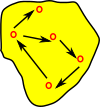Every element has 1 incoming arrow. May have zero or multiple outgoing arrows. Cop->setEvery element maps to a set.

## Example - Fibre Bundle as Database

 Here Cop is a database schema. This imposes a structure on the sets which are the database tables. This implements a category of simplical databases.Relational databases have many to one relationships between tables. For instance part of census data may have A table with an entry for each person. A table with an entry for each residence. There might then be a many to one relationship between these two tables. More information about relational databases on page here.In order to implement many to many relationships between tables we need to insert an extra table between them:## Fibre as a Pullback

#### Subset

If we take a subset of the function before inverting it we get a pullback.

 Function/morphism from A to C is given. Subset B of C is given. Hence we have two morphisms to C so this looks like the given part of a pullback.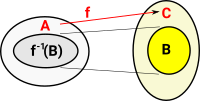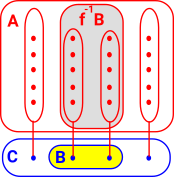So the structure in A relates to the structure in C. To complete the square we add cone for this diagram and we call it f-1(B) to emphasise that it is the inverse of 'f'.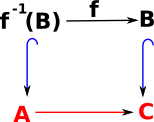#### Superset

 What if we do the opposite and take a superset of A rather than taking a subset of it? The function 'f' is only defined from A to C so we need to find some way to extend it to handle the bigger set. To do this we need to get some information about the bigger set by knowing something about the wider structure. For instance if we have a sheaf.More about pullbacks in catagory theory section on page here.

### Fibre With Structure

The examples so far show how reversing a map between sets gives some interesting structures.

The diagram on the right is intended to show a morphism between partial orders. So the blue arrows represent ≥and the arrow 'ab' means b ≥ a.

The red arrows show the morphism between the partial orders and these all need to play nicely together.So how can this morphism be reversed?

The diagram on the right is the best I can do although somehow it doesn't seem completely correct, for instance it seems to imply that c ≥ a whereas the original diagram above doesn't imply that.

In order to be consistent this morphism needs to be a local homeomorphism which is a function between topological spaces that preserves local but not necessarily global structure.

The interesting thing is that this is dividing the structure into 'local' structure and 'global' structure.### A fibre as a projection.

The simple case on the right is intended to show a projection of a three dimensional object onto a two dimensional surface. This is just a projection from a product space.

However a projection along a fibre is more general than that, locally it still looks like a product space, but globally it can look very different.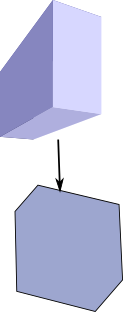A standard example of this is a helix, which can be projected onto a circle since any local section corresponds to a section of a circle. Locally a section of the spiral looks like a section of the circle, globally the circle and the spiral are different.

To reverse the direction of this projection we have to create a set (type) of points.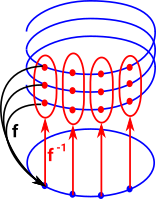A similar example is the Möbius band being mapped onto a cylinder.### Fibrations of Graphs

theory: topological graph theory

 undirected graph: covering projection directed graph: fibration (weaker form of covering projection)
 Here is an example for directed multigraphs. Each node in the top graph maps to the bottom graph (fibration). The corresponding node always has the same number and colour of incoming arcs (but not necessarily outgoing arcs). This gives some sort of local invariance.## Sheaves

Sheaves add extra structure to fibrations, so instead of just Set we now have:

CopSet

Where Cop gives the structure and Set holds the data.

This is known as a presheaf, to make this into a sheaf we need to be able to glue these elements of structure together.

## Next

sheaves

 metadata block see also: Correspondence about this page Book Shop - Further reading. Where I can, I have put links to Amazon for books that are relevant to the subject, click on the appropriate country flag to get more details of the book or to buy it from them.Introduction to Topological Manifolds (Graduate Texts in Mathematics S.) Other Books about Curves and Surfaces

This site may have errors. Don't use for critical systems.